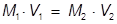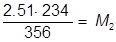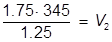# Dilution Formula

Dilution Formula

Dilution is a process in which the concentration of a solution is reduced. This is typically done by adding more solvent to the solution which decreases the number of moles per liter (M).M1 = the molarity of the original solution

V1 = the volume of the original solution

M2 = the molarity of the diluted solution

V2 = the volume of the diluted solution

Dilution Formula Questions:

1. If 234 mL of a 2.51 molar aqueous solution is diluted by adding water to give a final solution volume of 356 mL, what is the molarity of the diluted solution?

Note: The volume in this equation is typically meant for liters but because the units of mL will cancel it can be used.2. What volume of water must be added to 345 mL of a 1.75 M solution in order for it to be diluted to 1.25 M?The value of 483 mL is the final volume of the second solution, but does not answer the question. The volume of water that must be added is the difference between V1 and V2.

483 – 345 = 138 mL of water must be added